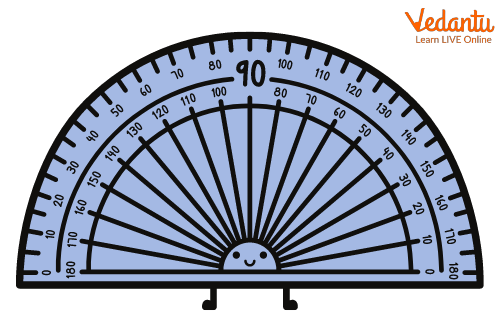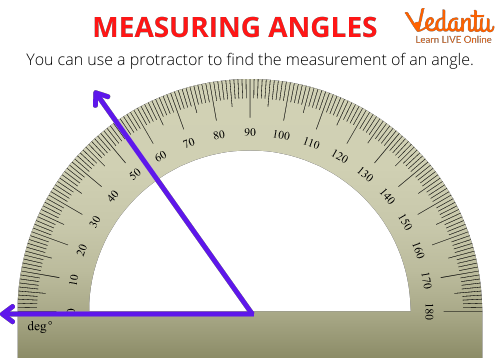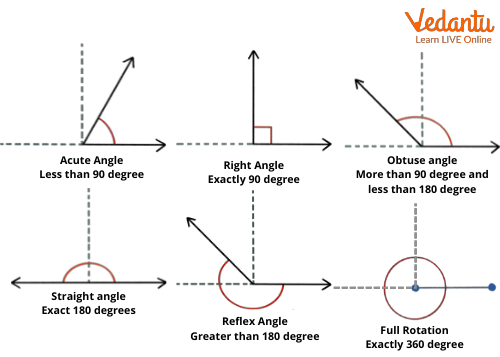Courses
Courses for Kids
Free study material
Free LIVE classes
More

# Angle MeasurementLIVE
Join Vedantu’s FREE Mastercalss

## Introduction to Angle Measurement

Before understanding the angle measurement methods kids must understand what an angle is. The angle is defined as the geometrical shape that is the resultant of the joining of two lines at their ends. Acute angle, obtuse angle, right angle, straight angle, reflex angle and full rotation angle are the angles that are taught to kids to help them better understand the concepts of geometry and as their grades progress the understanding of angles play a crucial role in the understanding of trigonometry. In this article, we are focused on providing the students with a short description of the common angles and the methods of angle measurement. Let us start our discussion by looking into how angles are measured?

### How is Angle Measurement Performed?

As we know angles are the geometrical shape that is formed by adjoining rays in an end to end fashion, this leads to the formation of inclination. The angle measurement is done in degrees. Now it is important for kids to note that this degree is the unit of measurement of angle. Kids must not confuse it with the measurement of the temperature. As the temperature is also measured in degrees Celsius and degrees Fahrenheit. The angle measurement is done with the help of a geometrical tool named a protractor.

### Protractor

As defined earlier protractor is defined as the tool used to measure the angles. The protractor is a semi-circle in shape. The tool is divided into equal parts which represent angles from 0 degrees to 180 degrees. It is important to note that a protractor has two readings, the inner and outer measurements are in opposite directions. The image mentioned below helps in a better understanding of the measurements of the protractor.Image illustrating the protractor measurement units

### Measuring Angle Using a Protractor

For measuring an angle using a protractor students must place the protractor on the vertex of the angle. The base of the proctor must be aligned with the base of the angle. The angle is then measured by counting from 0 up to the point where the ray intersects. It can be better understood by the diagram mentioned below.Measuring angle using the protractor

The image illustrates that the angle is 55 degrees.

Note- One of the most important points while performing angle measurement is to start the counting from zero degrees of the protractor scale. As in the example illustrated above, if the angle is measured by counting from 180 degrees, the angle measurement would have been 125 degrees, which is wrong.

### Types of Angles

Since we have learnt how to measure angles, let us look into the six types of angles.

Acute Angle

The angles that lie between 0 to 90 degrees are categorized as acute angles.

Obtuse Angle

The angle that lies between 90 to 180 degrees is said to be the obtuse angle, they are in a sense opposite to the acute angles.

Right Angle

The right angle is defined as 90 degrees. 91 degrees is considered an obtuse angle whereas 89 degrees is considered to be an acute angle.

Straight Angle

The angle that is 180 degrees is known as the straight angle.

Reflex Angle

An angle that is between 180 to 360 degrees is termed a reflex angle. It is greater than 180 degrees but is lesser than 360 degrees.

Full Rotation Angle

It is the angle whose measurement is 360 degrees, they are formed by the complete rotation of the ray. The image illustrated below represents all the angles discussed above.Image illustrating the different angles

In conclusion of the article, we have learnt about the angle measurement method using a protractor. We have also learnt about the various angles and their definitions. We hope that the study material provided will help students excel in geometry classes.

Last updated date: 20th Sep 2023
Total views: 137.7k
Views today: 3.37k

## FAQs on Angle Measurement

1. What is a vertex in geometry?

A vertex is defined as the point where two rays meet. Veratex plays a crucial role in angle measurement. The protractor must be aligned with the vertex of the angle for correct measurement. The two lines that arise from the vertex are called the arms of the angle.

2. What are positive and negative angles?

Angles are classified on the basis of rotation. In the case of the clockwise rotation from the base, the angle is termed as negative angle while if the rotation direction is in the anticlockwise direction the angle is said to be a positive angle.